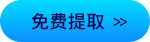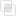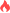php快速排序是什么意思2021-02-08 17:40:071020浏览 · 0收藏 · 0评论1.概念

2.排序步骤

（1）递归点：如果两个数组的元素大于1，就需要再进行分解

（2）递归出口：数组元素变成1的时候

3.实例

```<?php
// 快速排序
function quickOrder(\$arr)
{
// 判断是否需要运行,下面要拿出一个中间值
if (count(\$arr)<=1) {
return \$arr;
}
\$middle = \$arr; // 中间值
\$left   = array();
\$right  = array();
for (\$i = 1; \$i < count(\$arr); \$i++) {
if (\$middle < \$arr[\$i]) {
\$right[] = \$arr[\$i];
} else {
\$left[] = \$arr[\$i];
}
}
// 递归排序划分好的2边数组
\$left = quickOrder(\$left);
\$right = quickOrder(\$right);
return array_merge(\$left, array(\$middle), \$right);
}
\$arr = array(5, 2, 7, 6, 9, 3);
\$res = quickOrder(\$arr);
var_dump(\$res);```php数组排序函数php冒泡排序是什么意思php冒泡排序从小到大的操作php选择排序是什么php插入排序的用法python可变数据类型和不可变数据类型的区别274

Python3和Python2区别的整理967

Python解释器有哪几种310